# Math in Focus Grade 6 Chapter 8 Lesson 8.1 Answer Key Solving Algebraic Equations

Practice the problems of Math in Focus Grade 6 Workbook Answer Key Chapter 8 Lesson 8.1 Solving Algebraic Equations to score better marks in the exam.

## Math in Focus Grade 6 Course 1 B Chapter 8 Lesson 8.1 Answer Key Solving Algebraic Equations

### Math in Focus Grade 6 Chapter 8 Lesson 8.1 Guided Practice Answer Key

Complete each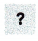with = or ≠, and each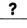with the correct value.

Question 1.
For what value of x will x + 3 = 7 be true?
If x = 1, x + 3 =+ 3
=(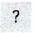7)
If x = 2, x + 3 =+ 3
=(7)
If x = 4, x + 3 =+ 3
=x + 3 = 7 is true when x =.
x + 3 = 7 is true when x = 4,

Explanation:
Given to find for what value of x will x + 3 = 7 be true, so
if x =1, x + 3 = 1 + 3 = 4 ≠ 7,
If x = 2, x + 3 = 2 + 3 = 5 ≠ 7,
if x = 3, x + 3 = 3 + 3 = 6 ≠ 7,
if x = 4, x + 3 = 4 + 3 = 7 = 7, therefore x + 3 = 7 is true when x = 4.

Solve each equation using the substitution method.

Question 2.
p + 6 = 13
p = 7, p + 6 = 13 is true,

Explanation:
Given equation p + 6 = 13, so solving p = 13 – 6 = 7, So when p = 7 means 7 + 6 = 13 is true therefore p = 7, p + 6 = 13 is true.

Question 3.
r + 4 = 12
r = 8, r + 4 = 12 is true,

Equation::
Given equation r + 4 = 12 so solving r = 12 – 4 = 8 therefore when r = 8 means 8 + 4 = 12 is true, therefore r = 8, r + 4 = 12 is true.

Question 4.
k – 10 = 7
k = 17 means k – 10 = 7 is true,

Explanation:
Given equation k – 10 = 7 so solving k = 7 + 10 = 17 therefore when k = 17 means 17 – 10 = 7 is true so k = 17., k – 10 = 7 is true.

Question 5.
2m = 6
m = 3 means 2m = 6 is true,

Explanation:
Given equation 2m = 6 so solving m =6/2 = 3 therefore when m = 3 means 2 x 3 = 6 is true so m = 3.

Question 6.
4n = 20
n = 5 means 4n = 20 is true,

Explanation:
Given equation 4n = 20 so solving n = 20/4 = 5 therefore when n = 5 means 4 X 5 = 20 is true so n = 5.

Question 7.
$$\frac{1}{5}$$z = 3
z = 15 means $$\frac{1}{5}$$z = 3,

Explanation:
Given equation $$\frac{1}{5}$$z = 3 so solving x = 3 X 5 = 15 therefore when z = 15 means $$\frac{1}{5}$$ X 15 = 3 is true so z = 3.
Complete each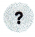with + or —, and each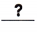with the correct value.

Question 8.
Solve x + 8 = 19.
x + 8 = 19
x + 8=x =x = 11,

Explanation:
Given to solve x + 8 = 19,
x + 8 – 19 = 19 – 19,
x – 11 = 0,
x = 11.

Solve each equation.

Question 9.
f + 5 = 14
f = 9,

Explanation:
Given to solve f + 5 = 14,
subtracting both sides by 12 as f + 5 – 14 = 14 – 14,
f – 9 = 0,
therefore f = 9.

Question 10.
26 = g + 11
g = 15,

Explanation:
Given to solve 26 = g + 11,
subtracting by 11 on the both sides as 26 – 11 = g + 11 – 11,
15 = g or g = 15.

Question 11.
w – 6 = 10
w = 16,

Explanation:
Given to solve w – 6 = 10,
Adding 6 both the sides we get w – 6 + 6 = 10 + 6,
therefore w = 16.

Question 12.
z – 9 = 21
z = 30,

Explanation:
Given to solve z – 9 = 21,
Adding 9 both the sides we get z – 9 + 9 = 21 + 9,
therefore z = 30.

Complete each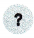with × or ÷, and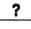with the correct value.

Question 13.
Solve 3x = 27.
3x = 27
3x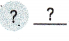= 27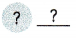x =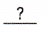x = 9,

Explanation:
Given to solve 3x = 27,
Dividing both sides by 3 as 3x ÷ 3 = 27 ÷ 3,
therefore x = 9.

Solve each equation.

Question 14.
6a = 42
a = 7,

Explanation:
Given to solve 6a = 42,
Dividing both sides by 6 as 6a ÷ 6 = 42 ÷ 6,
we get a = 7.

Question 15.
65 = 13b
b = 5,

Explanation:
Given to solve 65 = 13b,
Dividing both sides by 13 as 65 ÷ 13 = 13b ÷ 13,
we get 5 = b or b = 5.

Question 16.
$$\frac{m}{8}$$ = 9
m = 72,

Explanation:
Given to solve $$\frac{m}{8}$$ = 9,
Multiplying both sides by 8,
$$\frac{m}{8}$$ X 8 = 9 X 8,
We get m = 72.

Question 17.
12 = $$\frac{n}{7}$$
n = 84,

Explanation:
Given to solve 12 = $$\frac{n}{7}$$,
Multiplying both sides by 7,
12 X 7 = $$\frac{n}{7}$$ X 7,
we get 84 = n or n = 84.

Complete eachwith +, —, × or ÷, and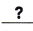with the correct value.

Question 18.
Solve x + $$\frac{3}{7}$$ = $$\frac{5}{7}$$
x + $$\frac{3}{7}$$ = $$\frac{5}{7}$$
x + $$\frac{3}{7}$$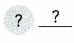= $$\frac{5}{7}$$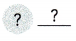x =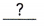x = $$\frac{2}{7}$$,

Explanation:
Given to solve x + $$\frac{3}{7}$$ = $$\frac{5}{7}$$ subtracting $$\frac{3}{7}$$ both sides as x + $$\frac{3}{7}$$ – $$\frac{3}{7}$$ = $$\frac{5}{7}$$ – $$\frac{3}{7}$$ we have common denominator so x = $$\frac{5 – 3}{7}$$ = $$\frac{2}{7}$$.

Solve each equation. First tell which operation you will perform on each side of the equation. Write your answer in simplest form.

Question 19.
k + $$\frac{1}{8}$$ = $$\frac{7}{8}$$
k = $$\frac{6}{8}$$ or $$\frac{3}{4}$$,

Explanation:
Given to solve k + $$\frac{1}{8}$$ = $$\frac{3}{4}$$ subtracting $$\frac{1}{8}$$ both sides as k + $$\frac{1}{8}$$ – $$\frac{1}{8}$$ = $$\frac{7}{8}$$ – $$\frac{1}{8}$$ we have common denominators so x = $$\frac{7 – 1}{8}$$ = $$\frac{6}{8}$$ further can be divided as both numerator and denominator goes by 2 we get $$\frac{3}{4}$$.

Question 20.
4p = $$\frac{3}{4}$$
p = $$\frac{3}{16}$$,

Explanation:
Given to solve 4p = $$\frac{3}{4}$$, Dividing both sides by 4 as
4p ÷ 4 = $$\frac{3}{4}$$ ÷ 4,
p = $$\frac{3}{4 X 4}$$ = $$\frac{3}{16}$$ .

### Math in Focus Course 1B Practice 8.1 Answer Key

Solve each equation using the substitution method.

Question 1.
b + 7 = 10
b = 3 means b + 7 = 10 is true,

Explanation:
Given equation b + 7 = 10 so solving b = 10 – 7 = 3 when b = 3 means 3 + 7 = 10 is true therefore b = 3.

Question 2.
17 = e + 9
e = 8,

Explanation:
Given 17 = e + 9 so solving e = 17 – 9 = 8 when e = 8 means 17 = 8 + 9  is true therefore e = 8.

Question 3.
k – 4 = 11
k = 15,

Explanation:
Given k – 4 = 11 so solving k = 11 + 4 = 15 when k = 15 means 15 – 4 = 11 is true therefore k = 15.

Question 4.
42 = 3p
p = 14,

Explanation:
Given 42 = 3p so solving 42/3 = p when p = 14 means 42 = 3 X 14 is true therefore p = 14.

Question 5.
8t = 56
t = 7,

Explanation:
Given 8t = 56 so solving t = 56/8 = 7 when t = 7 means 8 X 7 = 56 is true therefore t = 7.

Question 6.
$$\frac{1}{4}$$v = 5
v = 20,

Explanation:
Given $$\frac{1}{4}$$v = 5 solving v = 5 X 4 = 20 when v = 4 means $$\frac{1}{4}$$ X 4 = 5 x 4 = 20 is true therefore v = 4.

Solve each equation using the concept of balancing.

Question 7.
k + 12 = 23
k = 11,

Explanation:
Given to solve k + 12 = 23,
subtracting by 12 on the both sides as k + 12 – 12 = 23 – 12,
k = 11.

Question 8.
x- 8 = 17
x = 25,

Explanation:
Given to solve x – 8 = 17,
adding 8 by both sides as x – 8 + 8 = 17 + 8,
x = 25.

Question 9.
24 = f – 16
f = 40,

Explanation:
Given to solve 24 = f – 16,
adding both sides by 16 as 24 + 16 = f – 16 + 16,
40 = f or f =40.

Question 10.
5j = 75
j = 15,

Explanation:
Given to solve 5j = 75,
dividing both sides by 5 as 5j/5 = 75/5,
j = 15.

Question 11.
81 = 9m
m = 9,

Explanation:
Given to solve 81 = 9m,
dividing both sides by 9 as 81/9 = 9m/9,
9 = m or m = 9.

Question 12.
$$\frac{r}{6}$$ = 11
r = 66,

Explanation:
Given to solve $$\frac{r}{6}$$ = 11,
multiplying both sides by 6 as $$\frac{r}{6}$$ X 6 = 11 X 6,
r = 66.

Solve each equation using the concept of balancing. Write all fraction answers in simplest form.

Question 13.
$$\frac{5}{6}$$ = c + $$\frac{1}{6}$$
c = $$\frac{4}{6}$$ or $$\frac{2}{3}$$,

Explanation:
Given to solve $$\frac{5}{6}$$ = c + $$\frac{1}{6}$$ so subtracting both sides by $$\frac{1}{6}$$ we get $$\frac{5}{6}$$ – $$\frac{1}{6}$$ = c + $$\frac{1}{6}$$ – $$\frac{1}{6}$$ as we have common denominators we get $$\frac{5 – 1}{6}$$ = c,
c = $$\frac{4}{6}$$ we further divide as both numerator and denominators goes by 2 we get c = $$\frac{2 X 2}{3 X 2}$$, c = $$\frac{2}{3}$$.

Question 14.
h + $$\frac{5}{14}$$ = $$\frac{11}{14}$$
h = $$\frac{6}{14}$$ or $$\frac{3}{7}$$,

Explanation:
Given to solve h + $$\frac{5}{14}$$ = $$\frac{11}{14}$$ so subtracting both sides by $$\frac{5}{14}$$ we get h + $$\frac{5}{14}$$ – $$\frac{5}{14}$$ = $$\frac{11}{14}$$ – $$\frac{5}{14}$$ as we have common denominators we get h = $$\frac{11 – 5}{6}$$,
h = $$\frac{6}{14}$$ we further divide as both numerator and denominators goes by 2 we get h = $$\frac{3 X 2}{7 X 2}$$, h = $$\frac{3}{7}$$.

Question 15.
q – $$\frac{3}{10}$$ = $$\frac{7}{10}$$
q = 1,

Explanation:
Given to solve q – $$\frac{3}{10}$$ = $$\frac{7}{10}$$ so adding both sides by $$\frac{3}{10}$$ we get q – $$\frac{3}{10}$$ + $$\frac{3}{10}$$ = $$\frac{7}{10}$$ + $$\frac{3}{10}$$ as we have common denominators we get q = $$\frac{7 + 3}{10}$$,
q = $$\frac{10}{10}$$ we further divide as both numerator and denominators goes by 10 we get q = $$\frac{1 X 10}{1 X 10}$$, q = 1.

Question 16.
7k = $$\frac{4}{7}$$
k = $$\frac{4}{49}$$,

Explanation:
Given to solve 7k = $$\frac{4}{7}$$ dividing both sides by $$\frac{1}{7}$$ we get 7k X $$\frac{1}{7}$$ = $$\frac{4 X 1}{7 X 7}$$, k = $$\frac{4}{49}$$.

Question 17.
$$\frac{5}{12}$$ = 5d
d = $$\frac{1}{12}$$,

Explanation:
Given to solve $$\frac{5}{12}$$ = 5d so dividing both sides by $$\frac{1}{5}$$ we get $$\frac{5}{12}$$ X $$\frac{1}{5}$$ = 5d X $$\frac{1}{5}$$, d = $$\frac{1}{12}$$.

Question 18.
$$\frac{1}{2}$$x = $$\frac{1}{4}$$
x = $$\frac{1}{2}$$,

Explanation:
Given to solve $$\frac{1}{2}$$x = $$\frac{1}{4}$$ so multiplying both sides by 2 we get x X $$\frac{2}{2}$$ = $$\frac{2}{4}$$ we get x = $$\frac{2}{4}$$ we further divide as both numerator and denominators goes by 2 we get x = $$\frac{1 X 2}{2 X 2}$$, x = $$\frac{1}{2}$$.

Question 19.
$$\frac{8}{9}$$ = $$\frac{1}{3}$$f
f = $$\frac{8}{3}$$,

Explanation:
Given to solve $$\frac{8}{9}$$ = $$\frac{1}{3}$$f so multiplying both sides by 3 we get $$\frac{8}{9}$$ = $$\frac{1}{3}$$f X 3, $$\frac{8 X 3}{3 X 3}$$= f, So f = $$\frac{8}{3}$$.

Question 20.
r + 2.1 = 4.7
r = 2.6,

Explanation:
Given to solve r + 2.1 = 4.7 subtracting both sides by 2.1 we get r + 2.1 – 2.1 = 4.7 – 2.1, so r = 2.6.

Question 21.
9.9 = x + 5.4
x = 4.5,

Explanation:
Given to solve 9.9 = x + 5.4 subtracting both sides by 5.4 we get 9.9 – 5.4 = x – 5.4, so 4.5 = x.

Question 22.
11.2 = f – 1.8
f = 13,

Explanation:
Given to solve 11.2 = f – 1.8 adding both sides by 1.8 as 11.2 + 1.8 = f – 1.8 + 1.8 we get 13 = f or f = 13.

Question 23.
j – 3.7 = 20.4
j = 24.1,

Explanation:
Given to solve j – 3.7 = 20.4 adding both sides by 3.7 as j – 3.7 + 3.7 = 20.4 + 3.7 we get j = 24.1.

Question 24.
4w = 6.8
w = 1.7,

Explanation:
Given to solve 4w = 6.8, dividing both sides by 4 as 4w/4 = 6.8/4 we get w = 1.7.

Question 25.
13.9 = 2.5z
z = 5.56,

Explanation:
Given to solve 13.9 = 2.5z, dividing both sides by 2.5 as 13.9/2.5 = 2.5z/2.5 we get 5.56 = z or z = 5.56.

Question 26.
3.2d = 40.8
d = 12.75,

Explanation:
Given to solve 3.2d = 40.8 dividing both sides by 3.2 as 3.2d/3.2 = 40.8/3.2 we get d = 12.75.

Question 27.
x + $$\frac{1}{2}$$ = 1$$\frac{3}{4}$$
x = $$\frac{5}{4}$$ or 1$$\frac{1}{4}$$,

Explanation:
Given to solve x + $$\frac{1}{2}$$ = 1$$\frac{1}{4}$$ so subtracting both sides by $$\frac{1}{2}$$ we get x = $$\frac{1 X 4 + 3}{4}$$ – $$\frac{1}{2}$$ we get x = $$\frac{7}{4}$$ – $$\frac{1}{2}$$ , x = $$\frac{7 – 1 X 2}{4}$$ , x = $$\frac{5}{4}$$ as numerator is more we write in mixed fraction as x = $$\frac{1 X 4 + 1}{4}$$, x = 1$$\frac{1}{4}$$.

Question 28.
g + $$\frac{5}{3}$$ = 3$$\frac{2}{3}$$
g = 2,

Explanation:
Given to solve g + $$\frac{5}{3}$$ = 3$$\frac{2}{3}$$ so subtracting both sides by $$\frac{5}{3}$$ we get g = $$\frac{3 X 3 + 2}{3}$$ – $$\frac{5}{3}$$ we get g = $$\frac{11}{3}$$ – $$\frac{5}{3}$$ , g = $$\frac{11 – 5}{3}$$ , g = $$\frac{6}{3}$$ as numerator and denominator both goes by 3 we get g = $$\frac{2 X 3}{3 X 1}$$, g = 2.

Question 29.
2$$\frac{5}{7}$$ = p – $$\frac{2}{7}$$
p = 3,

Explanation:
Given to solve 2$$\frac{5}{7}$$ = p – $$\frac{2}{7}$$ so adding both sides by $$\frac{2}{7}$$ we get $$\frac{2 X 7 + 5}{7}$$ + $$\frac{2}{7}$$= p – $$\frac{2}{7}$$ + $$\frac{2}{7}$$, we have common denominators so $$\frac{19 + 2}{7}$$ = p, $$\frac{21}{7}$$ = p as numerator and denominator both goes by 7 we get p = $$\frac{7 X 3}{7 X 1}$$, p = 3.

Question 30.
e – $$\frac{18}{11}$$ = 1$$\frac{6}{11}$$
e = $$\frac{35}{11}$$ or 3$$\frac{2}{11}$$,

Explanation:
Given to solve e – $$\frac{18}{11}$$ = 1$$\frac{6}{11}$$ so adding both sides by $$\frac{18}{11}$$ we get e = $$\frac{11 X 1 + 6}{11}$$ + $$\frac{18}{11}$$ we get e = $$\frac{17}{11}$$ + $$\frac{18}{11}$$ both have common denominators we get e = $$\frac{17 + 18}{11}$$ , e = $$\frac{35}{11}$$ as numerator is greater than denominator we write in mixed fraction as e = $$\frac{3 X 11 + 2}{11}$$, so e = 3$$\frac{2}{11}$$.

Question 31.
$$\frac{4}{3}$$y = 36
y = 27,

Explanation:
Given to solve $$\frac{4}{3}$$y = 36 multiplying both sides by $$\frac{3}{4}$$ we get y = 36 X $$\frac{3}{4}$$, y = $$\frac{36 X 3}{4}$$ = $$\frac{4 X 9 X 3}{4}$$ we get y = 9 X 3 = 27.

Question 32.
$$\frac{9}{10}$$ = $$\frac{5}{6}$$v
v = $$\frac{54}{50}$$ or 1$$\frac{4}{50}$$,

Explanation:
Given to solve $$\frac{9}{10}$$ = $$\frac{5}{6}$$v multiplying both sides by $$\frac{6}{5}$$ we get $$\frac{9}{10}$$ X $$\frac{6}{5}$$ =v, v = $$\frac{9 X 6}{10 X 5}$$ = $$\frac{54}{50}$$ as numerator is greater we can further write in mixed fraction as $$\frac{1 X 50 + 4}{50}$$ = 1$$\frac{4}{50}$$.

Question 33.
$$\frac{2}{3}$$k = 28 ∙ $$\frac{4}{9}$$
k = $$\frac{56}{3}$$ or 18$$\frac{2}{3}$$,

Explanation:
Given to solve $$\frac{2}{3}$$k = 28 . $$\frac{4}{9}$$ multiplying both sides by $$\frac{3}{2}$$ we get k = $$\frac{28 X 4}{9}$$ X $$\frac{3}{2}$$, k = $$\frac{28 X 2}{3}$$, k = $$\frac{56}{3}$$ as numerator is greater we can further write in mixed fraction as $$\frac{18 X 3 + 2}{3}$$ = 18$$\frac{2}{3}$$.

Solve.

Question 34.
Find five pairs of whole numbers, such that when they are inserted into the equation below, the solution of the equation is 3,
x +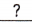=(0,3), (1,4), (2,5), (3,6) and (4,7),

Explanation:
Given to find five pairs of whole numbers such that when they are inserted into the equation x + __ =___  the solution of the equation is 3 so
1. When (0,3) substituting x + 0 = 3 yes true as x = 3,
2. When (1,4) substituting x + 1 = 4 solving we get x = 4 – 1 = 3 which is true,
3. When (2,5) substituting x + 2 = 5 solving we get x = 5 – 2 = 3 which is true,
4. When (3,6) substituting x + 3 = 6 solving we get x = 6 – 3 = 3 which is true,
5. When (4,7) substituting x + 4 = 7 solving we get x = 7 – 4 = 3 which is true therefore 5 pairs of whole numbers are (0,3), (1,4), (2,5), (3,6) and (4,7).

Question 35.
Find five pairs of numbers, such that when they are inserted into the equation below, the solution of the equation is $$\frac{2}{5}$$.x =($$\frac{2}{5}$$, 1), ($$\frac{1}{5}$$, 2), ($$\frac{2}{15}$$, 3), ($$\frac{2}{20}$$,4), ($$\frac{2}{25}$$, 5),
Given to find five pairs of numbers such that when they are inserted into the equation ___x = ____ , the solution of the equation is $$\frac{2}{5}$$ so
1. When ($$\frac{2}{5}$$,1) substituting $$\frac{2}{5}$$ X 1 = $$\frac{2}{5}$$,
2. When ($$\frac{1}{5}$$, 2) substituting $$\frac{1}{5}$$ X 2 = $$\frac{2}{5}$$,
3. When ($$\frac{2}{15}$$, 3) substituting $$\frac{2}{15}$$ X 3 = $$\frac{2}{5}$$,
4. When ($$\frac{2}{20}$$,4) substituting $$\frac{2}{20}$$ X 4 = $$\frac{2}{5}$$,
5. When ($$\frac{2}{5}$$,5) substituting $$\frac{2}{25}$$ X 5 = $$\frac{2}{5}$$ therefore 5 pairs of numbers are ($$\frac{2}{5}$$, 1), ($$\frac{1}{5}$$, 2), ($$\frac{2}{15}$$, 3), ($$\frac{2}{20}$$,4), ($$\frac{2}{25}$$, 5).